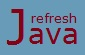# Java for each loop

If you have to traverse(iterate) an array/collection elements using normal for loop, you would have to write the `for` loop expressions like `initialization, condition, increment/decrement` which is a bit painful for programmers and sometimes incorrect declaration may also result as infinite loop. To simplify the traversal java introduces another looping statement known as `for-each` loop.

`for-each` loop is an enhanced form of normal `for` loop which is basically used to traverse elements of arrays or collection. It was introduced in java 5 just to simplify the traversing of arrays and collection elements. The Syntax of for-each loop is :

``` `for`(`DataType` item : array/collection variable )
{
`// Code to be executed once for each element in array/collection.`
} ```

Here `item` is the name of variable given by the programmer while the data type of this variable should be same or compatible with data type of array/collection variable. Array/collection variable is the array or collection variable whose elements need to be traverse. You should read the colon(:) as `"in"`, so the loop above is read as "for each item in array/collection variable".

### What is collection in java ?

Collection in java is a group of data structures(classes and interfaces). Variable of these data structures can hold multiple elements like arrays do. For example ArrayList, LinkedList, HashSet, HashMap etc are some of the collection data structures.

### Why it is called for-each loop ?

Because the loop iterates through each element of array/collection.

### When should I prefer for-each loop over normal for loop ?

When you just want to traverse each element of array/collection one by one.

### for each loop program in Java

```` class` ForEachLoop
{
`public static void` main(String [] args)
{
`int`[] marks = new `int`[]{40,60,80,65,70};
System.out.println(`"Accessing array element using normal for loop ........"`);
`for`(`int` i =0; i < marks.length; i++)
{
System.out.println(marks[i]);
}
System.out.println(`"Accessing array element using for-each loop ........"`);
`for`(`int` num : marks)
{
System.out.println(num);
}
}
}```

Output:

Accessing array element using normal for loop ........
40
60
80
65
70
Accessing array element using for-each loop ........
40
60
80
65
70

As you can see you don't have to write the expressions like `initialization, condition, increment/decrement` which is used in normal `for` loop and it's also easy to write and more readable as well. So you should prefer to use for-each loop over normal `for` loop while traversing array/ collection elements. You should read this as, "for each num in marks" execute the code inside the body.

## How for each loop works

1. It picks one element from array/collection
2. Assigns that element in variable given in left side
3. Executes the body of for-each loop
4. Then again moves to first step for the next element or finishes if all the elements are traversed.

## foreach vs for loop

1. You can not modify the elements of array/collection using for-each loop as you don't get the indexes while the same can be done in normal `for` loop. For example below thing can not be achieved using for-each loop.
``` `for`(`int` i = 0; i < marks.length-1; i++)
{
`if`(i==3)
{
marks[i] = 70;
}
} ```
2. You can traverse an array/collection from last to first using normal `for` loop as given below, but the same can not be achieved using for-each loop as it iterates only forward over the array/collection.
``` `for`(`int` i = marks.length-1; i>=0; i--)
{
System.out.println(marks[i]);
} ```
3. You can access multiple elements in a single iteration using `for` loop as given below, but the same can not be achieved using for-each loop.
``` `for`(`int` i = 0; i < marks.length-1; i++)
{
`if`(marks[i] == marks[i+1])
{
}
} ```
4. Prefer normal `for` loop over for-each loop whenever indexes is needed while iteration as for-each loop doesn't keep track of indexes.
★★★
• Using for each loop avoids occurrence of infinite loop.
• Using for each loop also ensures that your program won't throw ArrayIndexOutOfBoundsException while traversing the arrays.
• In for-each loop, `each` is not a keyword, it's just the name of looping statement.